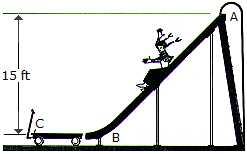# Engineering Mechanics - KOP: Impulse and Momentum - Discussion

### Discussion :: KOP: Impulse and Momentum - General Questions (Q.No.16)

16.A girl having a weight of 40 lb slides down the smooth slide onto the surface of a 20-lb wagon. Determine the speed of the wagon at the instant the girl stops sliding on it. If someone ties the wagon to the slide at B, determine the horizontal impulse the girl will exert at C in order to stop her motion. Neglect friction and assume that the girl starts from rest at the top of the slide, A.

 [A]. vwagon = 20.7 ft/s Imp = 38.6 lb-s [B]. vwagon = 31.1 ft/s Imp = 38.6 lb-s [C]. vwagon = 31.1 ft/s Imp = 57.9 lb-s [D]. vwagon = 20.7 ft/s Imp = 57.9 lb-s

Explanation:

No answer description available for this question.